# Introduction

The High-Low Method is a technique of cost accounting, which is used to split mixed costs into variable and fixed components.

It is essential to note that the High-Low method is not very popular as it relies on extreme values of the population and can distort the cost distribution. However, the technique is one of the fastest to outline an estimation when developing forecast models and trying out different approaches to the initial assumptions for the model.

# High-Low Method

When applying the High-Low method for our cost model, we start by calculating the Variable Cost per unit, via the following formula:Where:

• AC is Activity Cost, or the costs at certain activity level;
• AU is Activity Units, or the units at the same activity level.

Once we have calculated the Variable Costs (VC) per unit, we can now use it to calculate the Fixed Costs (FC). There are two ways to do that, either using the Highest Activity or the Lowest Activity:This way, we can arrive at a simple cost model:Remember that when figuring out the highest and lowest data points, we should not look at cost, but rather at unit volumes, as they are the driver behind the cost. What this means is that if we have a cost of 1,000 at a unit volume of 200 and a cost of 980 at 210 units, our High data point should be at 210 units, even if the value at 200 exceeds that.

# Example

Let us look at an example to understand better how to apply the High-Low method. We start with our reference data, which will be used to forecast costs for FY 2019. Therefore we have the Costs and the Units Volume of production for FY 2018 as a starting point.We can go ahead and calculate the High and Low points based on this data: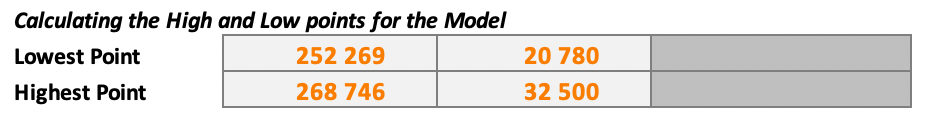However, doing this, we take the risk of including outliers in our analysis. Therefore it’s a better idea first to see if there are apparent outliers that we need to exclude. We do that by plotting the data points on a scatter diagram.By looking at the scatter diagram above, it is easy to spot that the high point [32 500; 268 746] is an outlier. If we calculate the Average Cost/Unit, we can also see that it significantly deviates from other months in the period.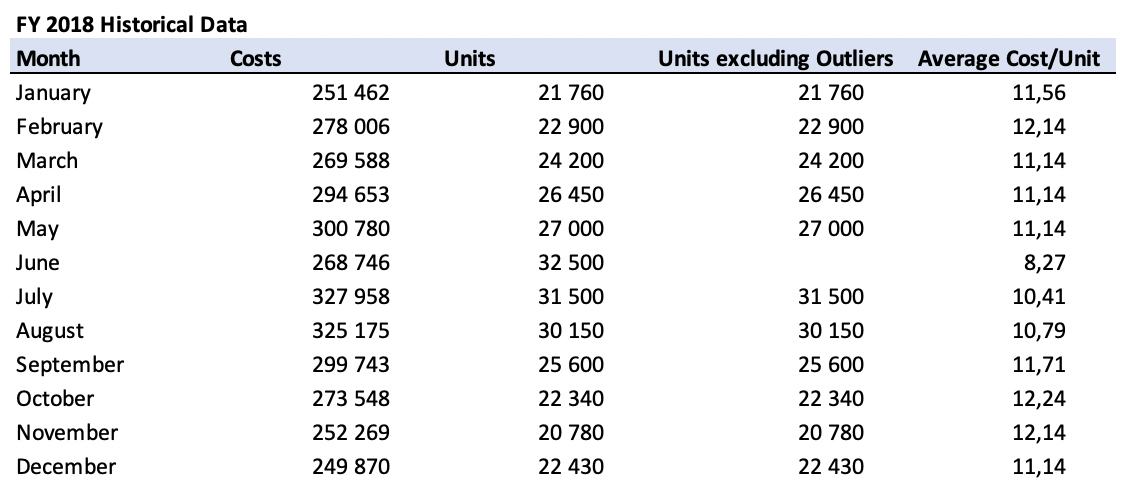Noticing such deviation is a reliable indicator that we should exclude the data point from our analysis. Identifying the high and low points now look like this.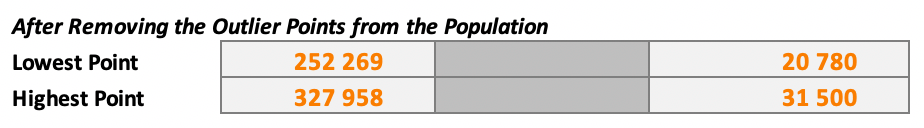Applying the High-Low method formula, we calculate the Variable Cost per unit.Following the method, we can calculate the Fixed Costs. Doing so via both the high and low data points is a good idea, as if these mismatch, we can identify possible technical errors in our calculations.This gives us our simple Cost Model: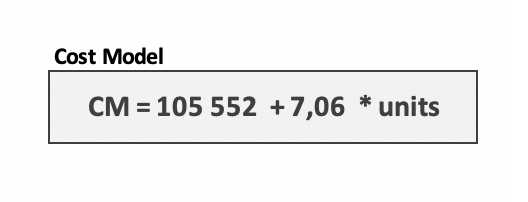Applying this to the forecasted unit volume for FY 2019, we can estimate the costs associated with the forecasted unit volumes.Comparing the FY 2019 forecast to the historical data for FY 2018 shows us that overall, we can expect a slight steady increase. The substantial deviation in June corresponds to where we identified our outlier.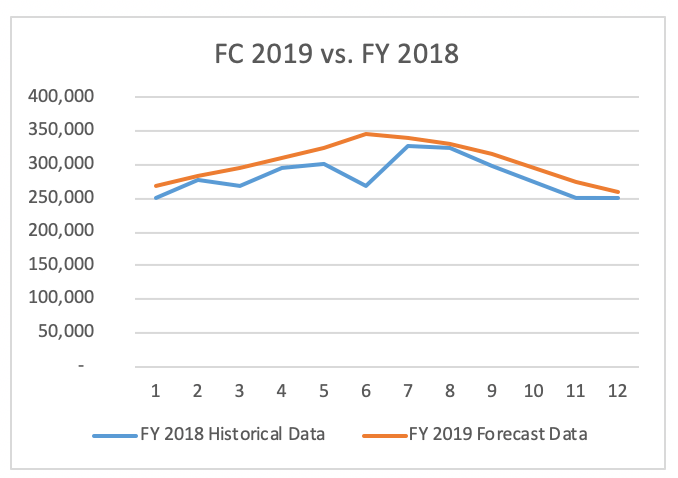The High-Low method has a lot of disadvantages and some more specific advantages:

(+) The High-Low method is an easy way to separate Fixed and Variable costs;

(+) It only requires two data points and basic math knowledge;

(+) It makes determining information about the cost behaviors quick;

(+) The method requires no special tools or programs;

(-) It does not take into consideration things such as cost variations;

(-) The High-Low method assumes Variable and Fixed costs are constant throughout different activity levels, which is usually not the case in reality;

(-) It is by far not the best option out there, and there are much better alternatives like the Least-Squares Regression Model that consider all data points in the population and provide much better results.

(-) One of the High/Low points, or both, might not be representative of the costs usually incurred at those unit volume levels. We can mitigate this risk by calculating the model with more data points to confirm the relationship between the costs. This may lead us to conclude that a point is an outlier, and we can then exclude it from our analysis, to get a more reliable cost model.

(-) The High-Low method does not consider costs that don’t change proportionally with unit volume changes, but rather at discrete points, also known as Step Costs. If there’s a step cost between the Low and High points, this will be incorrectly attributed entirely to variable costs, while in reality, it can be a step cost either in Fixed or Variable costs.

# Conclusion

The High-Low method is particularly useful with pricing analysis and forecasting for budgets, as it can be used with any mixed cost, be it associated with a product, product group, warehouse, customer, or others.

One of the main advantages of the High-Low method is that it’s a straightforward method to analyze cost without getting into complex calculations.

However, it is essential to remember that the method is an estimate, and we should only use it in corroboration with other sources of information where possible.

Due to all its limitations, the High-Low method is not widely used, but it remains one of the simplest ways to prepare fast and rough forecasts.

Thank you for reading and don’t forget to take a look at our example Excel file below:## FCCA, FMVA

Hi! I am a finance professional with 10+ years of experience in audit, controlling, reporting, financial analysis and modeling. I am excited to delve deep into specifics of various industries, where I can identify the best solutions for clients I work with.

In my spare time, I am into skiing, hiking and running. I am also active on Instagram and YouTube, where I try different ways to express my creative side.

The information and views set out in this publication are those of the author(s) and do not necessarily reflect the official opinion of Magnimetrics. Neither Magnimetrics nor any person acting on their behalf may be held responsible for the use which may be made of the information contained herein. The information in this article is for educational purposes only and should not be treated as professional advice. Magnimetrics and the author of this publication accept no responsibility for any damages or losses sustained in the result of using the information presented in the publication.